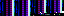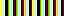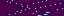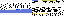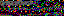```from pygame import display
from pygame.font import Font
from pygame.time import get_ticks, wait

from GameChild import GameChild

class Mainloop(GameChild):

def __init__(self, parent):
GameChild.__init__(self, parent)
self.overflow = 0
self.frame_count = 1
self.actual_frame_duration = 0
self.frames_this_second = 0
self.last_framerate_display = 0
self.init_framerate_display()
self.last_ticks = get_ticks()
self.stopping = False

config = self.get_configuration("display")
self.target_frame_duration = config["frame-duration"]
self.wait_duration = config["wait-duration"]
self.skip_frames = config["skip-frames"]
self.show_framerate = config["show-framerate"]
self.framerate_text_size = config["framerate-text-size"]
self.framerate_text_color = config["framerate-text-color"]
self.framerate_text_background = config["framerate-text-background"]
self.framerate_display_flag = config["framerate-display-flag"]

def init_framerate_display(self):
if self.framerate_display_active():
screen = self.get_screen()
self.last_framerate_count = 0
self.framerate_topright = screen.get_rect().topright
self.display_surface = screen
self.font = Font(None, self.framerate_text_size)
self.font.set_bold(True)
self.render_framerate()

def framerate_display_active(self):
return self.check_command_line(self.framerate_display_flag) or \
self.show_framerate

def render_framerate(self):
text = self.font.render(str(self.last_framerate_count), False,
self.framerate_text_color,
self.framerate_text_background)
rect = text.get_rect()
rect.topright = self.framerate_topright
self.framerate_text = text
self.framerate_text_rect = rect

def run(self):
while not self.stopping:
self.update_frame_duration()
self.update_overflow()
self.stopping = False

refresh = False
while self.frame_count > 0:
refresh = True
self.parent.frame()
if self.framerate_display_active():
self.update_framerate()
self.frame_count -= 1
if not self.skip_frames:
break
if refresh:
display.update()

def update_frame_duration(self):
last_ticks = self.last_ticks
actual_frame_duration = get_ticks() - last_ticks
last_ticks = get_ticks()
while actual_frame_duration < self.target_frame_duration:
wait(self.wait_duration)
actual_frame_duration += get_ticks() - last_ticks
last_ticks = get_ticks()
self.actual_frame_duration = actual_frame_duration
self.last_ticks = last_ticks

def update_overflow(self):
self.frame_count = 1
target_frame_duration = self.target_frame_duration
overflow = self.overflow
overflow += self.actual_frame_duration - target_frame_duration
while overflow > target_frame_duration:
self.frame_count += 1
overflow -= target_frame_duration
overflow = self.overflow

def update_framerate(self):
count = self.frames_this_second + 1
if get_ticks() - self.last_framerate_display > 1000:
if count != self.last_framerate_count:
self.last_framerate_count = count
self.render_framerate()
self.last_framerate_display = get_ticks()
count = 0
self.display_surface.blit(self.framerate_text, self.framerate_text_rect)
self.frames_this_second = count

def stop(self):
self.stopping = True
```
```from os import makedirs
from os.path import exists, join
from sys import exc_info
from time import strftime

from pygame import image

from GameChild import *
from Input import *

class ScreenGrabber(GameChild):

def __init__(self, game):
GameChild.__init__(self, game)
self.delegate = self.get_delegate()
self.subscribe(self.save_display)

config = self.get_configuration("screen-captures")
self.save_path = config["path"]
self.file_name_format = config["file-name-format"]
self.file_extension = config["file-extension"]

def save_display(self, event):
if self.delegate.compare(event, "capture-screen"):
directory = self.save_path
try:
if not exists(directory):
makedirs(directory)
name = self.build_name()
path = join(directory, name)
capture = image.save(self.get_screen(), path)
self.print_debug("Saved screen capture to %s" % (path))
except:
self.print_debug("Couldn't save screen capture to %s, %s" %\
(directory, exc_info()))

def build_name(self):
return "{0}.{1}".format(strftime(self.file_name_format),
self.file_extension)
```
```from random import randint
from math import sin, cos, atan2, radians, sqrt

def get_step(start, end, speed):
x0, y0 = start
x1, y1 = end
angle = atan2(x1 - x0, y1 - y0)
return speed * sin(angle), speed * cos(angle)

def get_endpoint(start, angle, magnitude):
"""clockwise, 0 is up"""
x0, y0 = start
dx, dy = get_delta(angle, magnitude)
return x0 + dx, y0 + dy

def get_delta(angle, magnitude):
return sin(angle) * magnitude, -cos(angle) * magnitude

def rotate_2d(point, center, angle, translate_angle=True):
if translate_angle:
x, y = point
cx, cy = center
return cos(angle) * (x - cx) - sin(angle) * (y - cy) + cx, \
sin(angle) * (x - cx) + cos(angle) * (y - cy) + cy

angle_step = 360.0 / count
points = []
current_angle = 0
for _ in xrange(count):
current_angle + offset))
current_angle += angle_step
return points

if translate_angle:

def get_range_steps(start, end, count):
for ii in xrange(count):
yield start + (end - start) * ii / float(count - 1)

def get_distance(p0, p1):
return sqrt((p0 - p1) ** 2 + (p0 - p1) ** 2)

def place_in_rect(rect, incoming, contain=True, *args):
while True:
incoming.center = randint(0, rect.w), randint(0, rect.h)
if not contain or rect.contains(incoming):
collides = False
for inner in args:
if inner.colliderect(incoming):
collides = True
break
if not collides:
break

def get_intersection(p0, p1, p2, p3):
x0, y0 = p0
x1, y1 = p1
x2, y2 = p2
x3, y3 = p3
a0 = y1 - y0
b0 = x0 - x1
c0 = x1 * y0 - x0 * y1
r2 = a0 * x2 + b0 * y2 + c0
r3 = a0 * x3 + b0 * y3 + c0
if r2 != 0 and r3 != 0 and r2 * r3 > 0:
return None
a1 = y3 - y2
b1 = x2 - x3
c1 = x3 * y2 - x2 * y3
r0 = a1 * x0 + b1 * y0 + c1
r1 = a1 * x1 + b1 * y1 + c1
if r0 != 0 and r1 != 0 and r0 * r1 > 0:
return None
denominator = a0 * b1 - a1 * b0
if denominator == 0:
return (x0 + x1 + x2 + x3) / 4, (y0 + y1 + y2 + y3) / 4
if denominator < 0:
offset = -denominator / 2
else:
offset = denominator / 2
numerator = b0 * c1 - b1 * c0
x = ((-1, 1)[numerator < 0] * offset + numerator) / denominator
numerator = a1 * c0 - a0 * c1
y = ((-1, 1)[numerator < 0] * offset + numerator) / denominator
return x, y

def collide_line_with_rect(rect, p0, p1):
for line in ((rect.topleft, rect.topright),
(rect.topright, rect.bottomright),
(rect.bottomright, rect.bottomleft),
(rect.bottomleft, rect.topleft)):
if get_intersection(p0, p1, *line):
return True
```
44.212.99.248
44.212.99.248
44.212.99.248

May 17, 2018

Line Wobbler Advance is a demake of Line Wobbler for Game Boy Advance that started as a demo for Synchrony. It contains remakes of the original Line Wobbler levels and adds a challenging advance mode with levels made by various designers.

f1. Wobble at home or on-the-go with Line Wobbler Advance

This project was originally meant to be a port of Line Wobbler and kind of a joke (demaking a game made for even lower level hardware), but once the original levels were complete, a few elements were added, including a timer, different line styles and new core mechanics, such as reactive A.I.

I reverse engineered the game by mapping the LED strip on paper and taking notes on each level. Many elements of the game are perfectly translated, such as enemy and lava positions and speeds and the sizes of the streams. The boss spawns enemies at precisely the same rate in both versions. Thanks in part to this effort, Line Wobbler Advance was awarded first prize in the Wild category at Synchrony.f3. First prize at Synchrony

Advance mode is a series of levels by different designers implementing their visions of the Line Wobbler universe. This is the part of the game that got the most attention. It turned into a twitchy gauntlet filled with variations on the core mechanics, cinematic interludes and new elements, such as enemies that react to the character's movements. Most of the levels are much harder than the originals and require a lot of retries.

Thanks Robin Baumgarten for giving permission to make custom levels and share this project, and thanks to the advance mode designers Prashast Thapan, Charles Huang, John Rhee, Lillyan Ling, GJ Lee, Emily Koonce, Yuxin Gao, Brian Chung, Paloma Dawkins, Gus Boehling, Dennis Carr, Shuichi Aizawa, Blake Andrews and mushbuh!